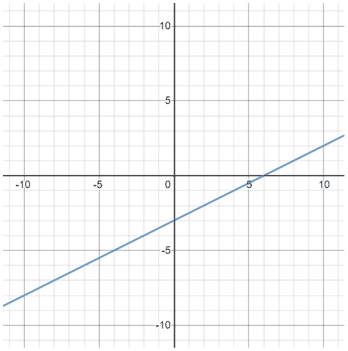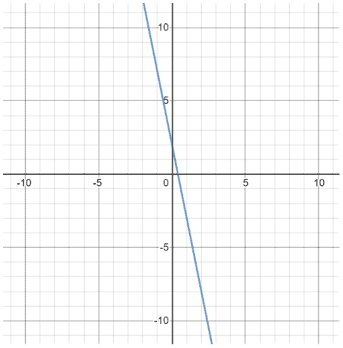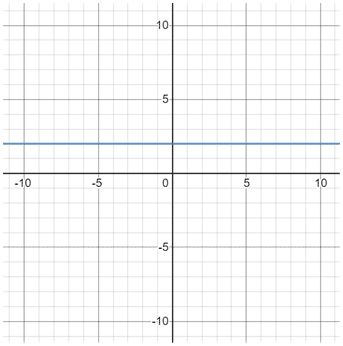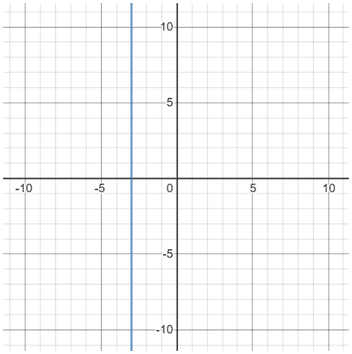# Slope

Slope is a value that describes the steepness and direction of a line. In variable format, it is commonly represented by the letter m. The slope of a line is also called its gradient or rate of change.

The slope formula is the vertical change in y divided by the horizontal change in x, sometimes called rise over run. The slope formula uses two points, (x1, y1) and (x2, y2), to calculate the change in y over the change in x.

Slope is a ratio that includes how y changes for every unit increase of x:A graphical depiction is shown below.

Below is an example of using the slope formula.

Example

Given the following points:

(-2, 3) and (4, 1)As the magnitude of the slope increases, the line becomes steeper. As the magnitude of the slope decreases, the opposite occurs, and the line becomes less steep.

For linear equations in slope-intercept form, y = mx + b, m indicates the slope of the line.

Slope also indicates the direction of a line. A line with a positive slope, said to be increasing, runs upwards from left to right.

A line with a negative slope, said to be decreasing, runs downwards from left to right.

Example

 Positive slope Negative slopeA horizontal line has a slope of zero because y does not change. A vertical line has an undefined slope because you cannot divide by zero (x does not change).

Example

 Zero slope Undefined slope y = 2 x = -3Parallel lines have the same slope.

Example

y = 2x + 3 and y = 2x - 4 both have a slope of 2, so they are parallel, as shown below:

Perpendicular lines have slopes that are "opposite reciprocals" of each other. In this context, "opposite" refers to the change in sign from + to - or vice versa. "Reciprocal" refers to flipping the numerator and denominator of the value. For example, the reciprocal of x is.

Therefore, taking the opposite reciprocal of something means that you flip the sign and the numerator and denominator.

Example

-3x - 2 has a slope of -3, and y = ⅓x + 1 has a slope of . -3 and are opposite reciprocals, so the equations are perpendicular: